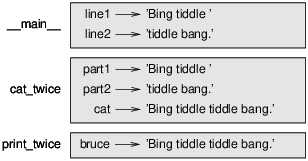# 3.9: Stack diagrams

$$\newcommand{\vecs}{\overset { \rightharpoonup} {\mathbf{#1}} }$$ $$\newcommand{\vecd}{\overset{-\!-\!\rightharpoonup}{\vphantom{a}\smash {#1}}}$$$$\newcommand{\id}{\mathrm{id}}$$ $$\newcommand{\Span}{\mathrm{span}}$$ $$\newcommand{\kernel}{\mathrm{null}\,}$$ $$\newcommand{\range}{\mathrm{range}\,}$$ $$\newcommand{\RealPart}{\mathrm{Re}}$$ $$\newcommand{\ImaginaryPart}{\mathrm{Im}}$$ $$\newcommand{\Argument}{\mathrm{Arg}}$$ $$\newcommand{\norm}{\| #1 \|}$$ $$\newcommand{\inner}{\langle #1, #2 \rangle}$$ $$\newcommand{\Span}{\mathrm{span}}$$ $$\newcommand{\id}{\mathrm{id}}$$ $$\newcommand{\Span}{\mathrm{span}}$$ $$\newcommand{\kernel}{\mathrm{null}\,}$$ $$\newcommand{\range}{\mathrm{range}\,}$$ $$\newcommand{\RealPart}{\mathrm{Re}}$$ $$\newcommand{\ImaginaryPart}{\mathrm{Im}}$$ $$\newcommand{\Argument}{\mathrm{Arg}}$$ $$\newcommand{\norm}{\| #1 \|}$$ $$\newcommand{\inner}{\langle #1, #2 \rangle}$$ $$\newcommand{\Span}{\mathrm{span}}$$$$\newcommand{\AA}{\unicode[.8,0]{x212B}}$$

To keep track of which variables can be used where, it is sometimes useful to draw a stack diagram. Like state diagrams, stack diagrams show the value of each variable, but they also show the function each variable belongs to.

Each function is represented by a frame. A frame is a box with the name of a function beside it and the parameters and variables of the function inside it. The stack diagram for the previous example is shown in Figure $$\PageIndex{1}$$.Figure $$\PageIndex{1}$$: Stack diagram.

The frames are arranged in a stack that indicates which function called which, and so on. In this example, print_twice was called by cat_twice, and cat_twice was called by __main__, which is a special name for the topmost frame. When you create a variable outside of any function, it belongs to __main__.

Each parameter refers to the same value as its corresponding argument. So, part1 has the same value as line1, part2 has the same value as line2, and bruce has the same value as cat.

If an error occurs during a function call, Python prints the name of the function, the name of the function that called it, and the name of the function that called that, all the way back to __main__.

For example, if you try to access cat from within print_twice, you get a NameError:

Traceback (innermost last):
File "test.py", line 13, in __main__
cat_twice(line1, line2)
File "test.py", line 5, in cat_twice
print_twice(cat)
File "test.py", line 9, in print_twice
print(cat)
NameError: name 'cat' is not defined


This list of functions is called a traceback. It tells you what program file the error occurred in, and what line, and what functions were executing at the time. It also shows the line of code that caused the error.

The order of the functions in the traceback is the same as the order of the frames in the stack diagram. The function that is currently running is at the bottom.

This page titled 3.9: Stack diagrams is shared under a CC BY-NC 3.0 license and was authored, remixed, and/or curated by Allen B. Downey (Green Tea Press) .